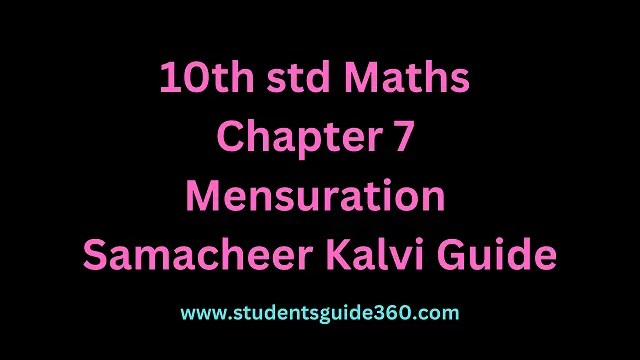# 10th Maths Chapter 7 Mensuration Exercise 7.3

10th Standard Maths Chapter 7 Mensuration Exercise 7.3 Guide. TN SSLC Samacheer Kalvi Guide Chapter 7 Exercise 7.3 Book Back Answers & Solutions. 10th All Subject Guide – Click Here. Class 1 to 12 All Subject Book Back Answers – Click Here.### 1. A vessel is in the form of a hemispherical bowl mounted by a hollow cylinder. The diameter is 14 cm and the height of the vessel is 13 cm. Find the capacity of the vessel.

Solution:
Diameter = 14 cm
Total height = 13 cm
Height of the cylindrical part = 13 – 7
= 6 cm
∴ Capacity of the vessel = Capacity of the cylinder + Capacity of the hemisphere.∴ The total volume = 924 + 718.67
The capacity of the vessel = 1642.67 cm3

### 2. Nathan, an engineering student was asked to make a model shaped like a cylinder with two cones attached at its two ends. The diameter of the model is 3 cm and its length is 12 cm. If each cone has a height of 2 cm, find the volume of the model that Nathan made.

Solution:
Volume of the model = Volume of the cylinder + Volume of 2 cones.The volume of the model that Nathan made = 66 cm3### 3. From a solid cylinder whose height is 2.4 cm and the diameter 1.4 cm, a cone of the same height and same diameter is carved out. Find the volume of the remaining solid to the nearest cm3.

Solution:
Volume of the cylinder = πr2h cu. units
Volume of the cone = 13πr2h cu. unitsd = 1.4 cm, r = 0.7 cm = 710
h = 2.4 cm = 2410
Volume of the cylinder:Volume of cone carved out∴ Volume of the remaining solid = Volume of the cylinder – Volume of the cone
= 3.696 – 1.232
= 2.464
= 2.46 cm3

### 4. A solid consisting of a right circular cone of height 12 cm and radius 6 cm standing on a hemisphere of radius 6 cm is placed upright in a right circular cylinder full of water such that it touches the bottom. Find the volume of the water displaced out of the cylinder, if the radius of the cylinder is 6 cm and height is 18 cm.Solution:
Volume of water displaced out = Volume of the solid immersed in.
Volume of the solid = Volume of the cone + Volume of the hemisphere∴ The volume of water displaced out = Volume of the solid
= (1) + (2)
= 905.14 cm3### 5. A capsule is in the shape of a cylinder with two hemispheres stuck to each of its ends. If the length of the entire capsule is 12 mm and the diameter of the capsule is 3 mm, how much medicine it can hold?

Solution:
Volume of medicine the capsule can hold = Volume of the cylinder + 2 volume of hemisphere
Volume of the cylinder part∴ The total volume = 56.571 mm3
∴ The volume of the medicine the capsule can hold = 56.57 mm3

### 6. As shown in the figure a cubical block of side 7 cm is surmounted by a hemisphere. Find the surface area of the solid.Solution:
Clearly, greatest diameter of the hemisphere is equal to the length of an edge of the cube is 7 cm.
Radius of the hemisphere = 72 cm
Now, total surface area of the solid = Surface area of the cube + Curved surface area of the hemisphere – Area of the base of the hemisphere.### 7. A right circular cylinder just enclose a sphere of radius r units.

Calculate

• (i) the surface area of the sphere
• (ii) the curved surface area of the cylinder
• (iii) the ratio of the areas obtained in (i) and (ii).

Solution:
(i) Surface area of sphere = 4πr2 sq. units### 8. A shuttle cock used for playing badminton has the shape of a frustum of a cone is mounted on a hemisphere. The diameters of the frustum are 5 cm and 2 cm. The height of the entire shuttle cock is 7 cm. Find its external surface area.

Solution:
The external surface area of the cock = Surface area of frustum + CSA of the hemisphere